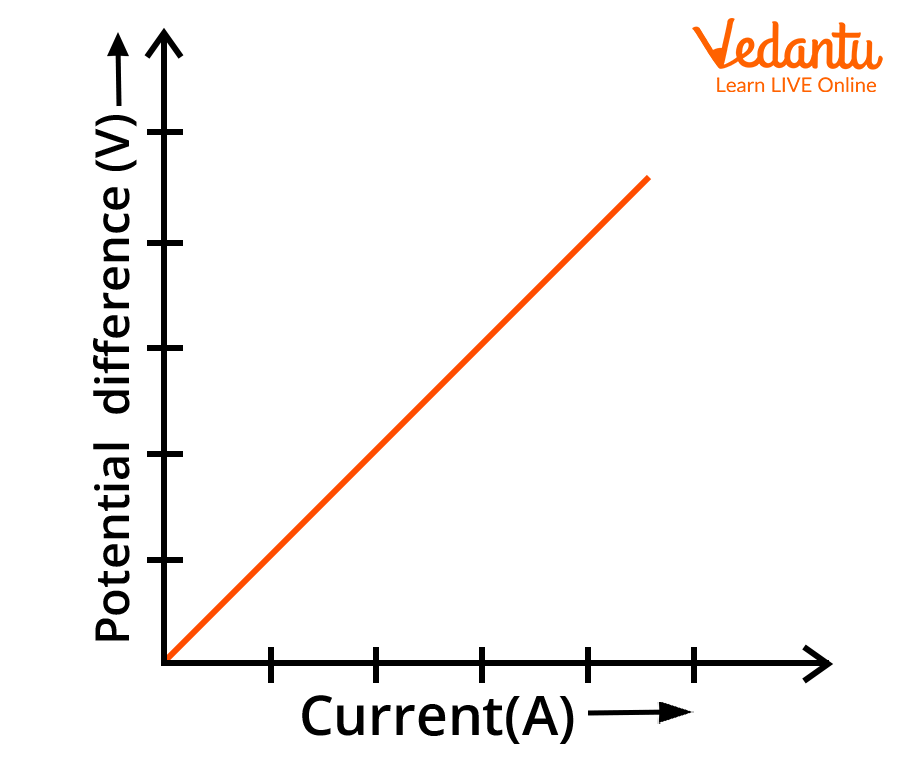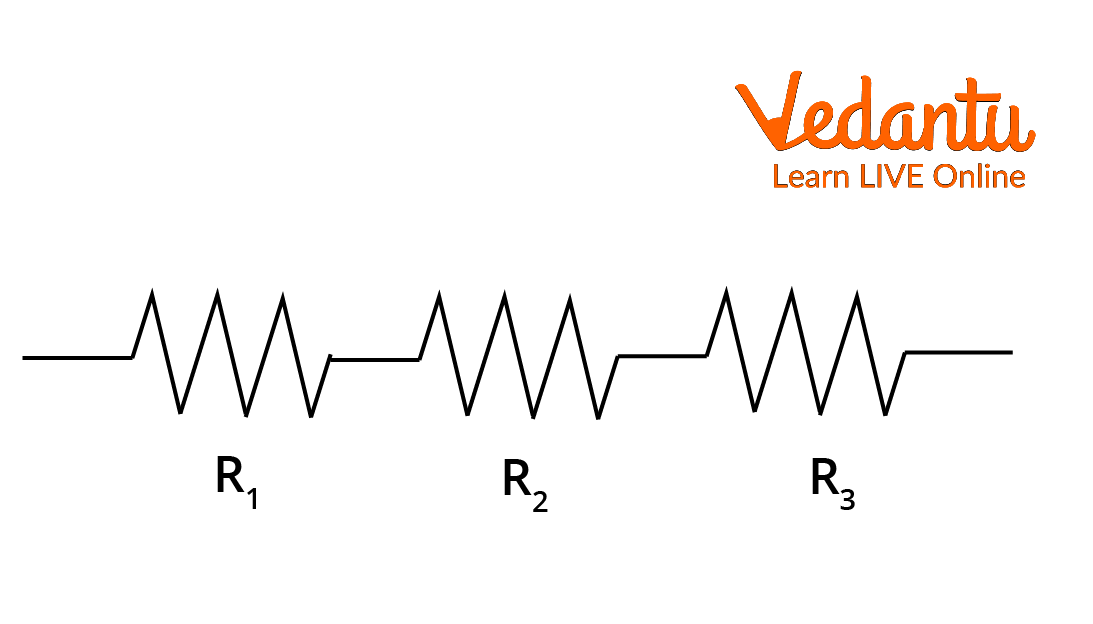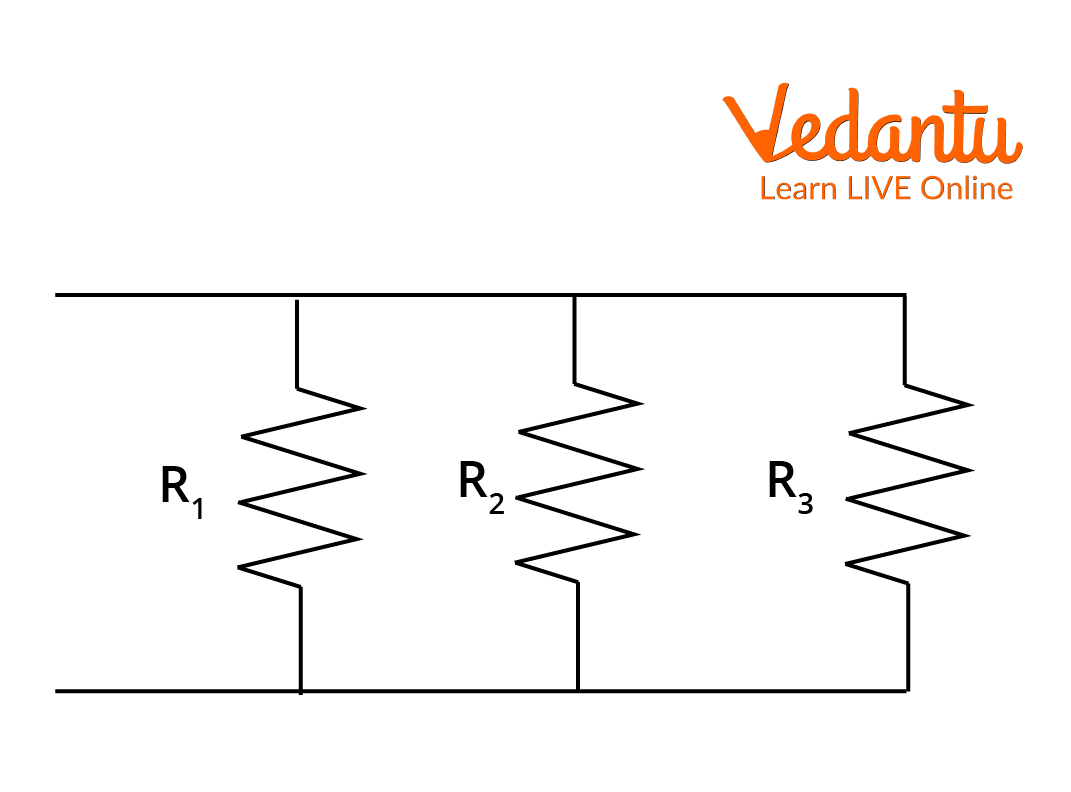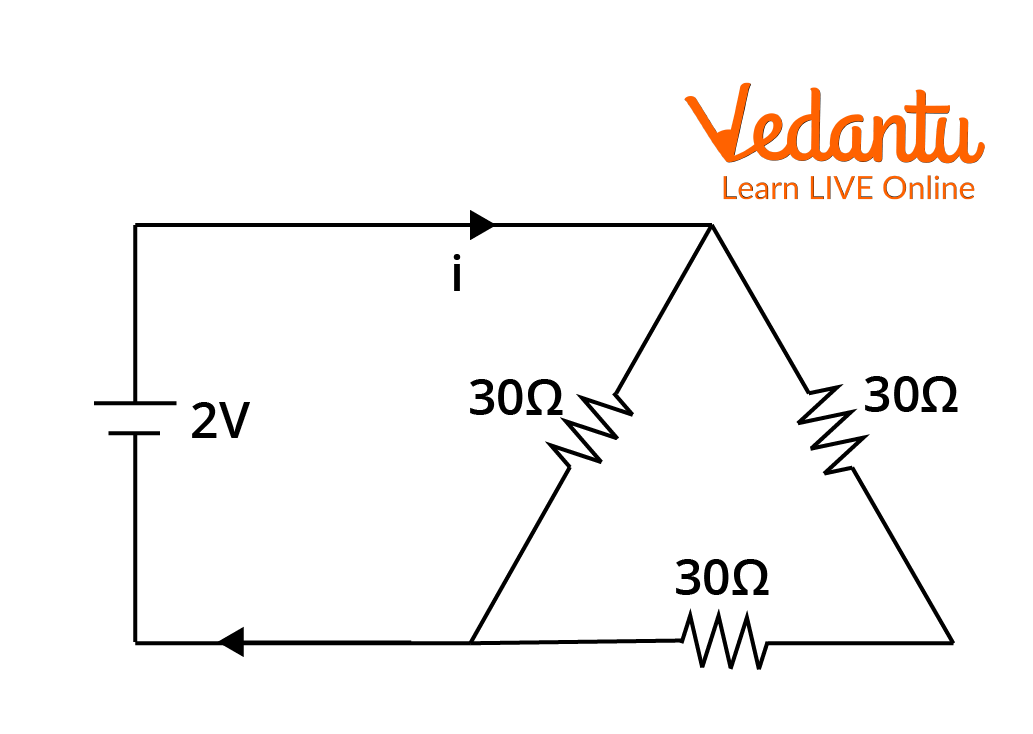Courses
Courses for Kids
Free study material
Free LIVE classes
More

# Electric Current and Potential Difference for JEELIVE
Join Vedantu’s FREE Mastercalss

## Insights into Current and Potential Difference

To understand the concept of potential difference, we need to be thorough with the concept of electric potential. The electric potential is a term that is used for the work done to move a unit electric charge from one position to another in an electrostatic field. The value of the electric potential at any point in an electric field is a scalar quantity. This is widely used to describe the operations going on in an electrostatic field in a simpler way.

This is measured in volts, and the difference between electric potential at one point to another point is termed the potential difference. This is often used and termed voltage in Physics. If a voltage flows in a circuit, it surely results in the movement of charges from one point to another inside the electric material. Therefore, the flow of charges in a cross-sectional area with respect to time is defined as electric current. This is measured in amperes. Let’s look more deeply into understanding the concept of electric current and potential difference.

## Relationship Between Current and Potential Difference

Let us see how the potential difference is calculated in a system of charges in an electrostatic field. To find the potential difference, let us start by finding the work done to move a point charge from position 1 to position 2.

### Potential Difference

Say, a test positive charge q is assumed at position 1 and the electrostatic force needed to pull it against the field is

$F_{e x}=-q \vec{E}$

where Fex is the external force needed to pull the charge against the electric field present in the material, i.e., E. As shown in the below figure, the external force is needed to apply to the test charge to move it against the electrostatic forces acting on it from position 1 to position 2 denoted by vector dl.Work done on a Test Charge to move from Position 1 to 2

So, the work done by any external force is given by,

$W_{e x}=\int_{1}^{2} \vec{F}_{e x} \vec{d} l=\int_{1}^{2}(-q \vec{E}) \vec{d} l$

Therefore, the work done by the external force is written as:

$W_{e x}=-q \int_{1}^{2} \vec{E} \cdot \vec{d} l$

In this equation, if we take some point existing at infinity as the position 1 of the test charge and let us assume point R as position 2 of the test charge, then we obtain

$W_{e x}=-q \int_{\infty}^{R} \vec{E} \cdot \vec{d} l$

Let us suppose the potential at point R is V and it can be derived according to the definition of work done per unit test charge to move from infinity to point R.

i.e., $V=\dfrac{W_{e x}}{q}=\int_{\infty}^{R} \vec{E} \cdot \vec{d} l$

This is the expression for electric potential in a static electric field.

The difference between potentials taken at two different points is termed potential difference. Let us look into the electric current flowing through a circuit in detail.

### Electric Current

The electric potential, or the voltage, results in the flow of charges, namely electrons, in the circuit, which results in the flow of current through the circuit. We write the current equation as the rate of flow of charges passing through a cross-section of area varying with time.

i.e.,  $I=\dfrac{d q}{d t}$

There are some important points to be noted about how the current flows inside the material. If there are both negative and positive charges flowing in the material, the direction of the flow of the current is opposite to the direction of the flow of electrons. That is in the direction of positive charges. Units of current are Amperes (A) and now let us look into the relationship between electric current and potential difference with the help of a graph between electric current and potential difference.

## The Graph Between Electric Current and Potential Difference

The relationship between electric current and the potential difference is given by Ohm’s law which states that in any conductor, the flowing electric current is directly proportional to the potential difference between the two ends of the conductor.

i.e., $V\propto I$

Therefore, V = IR where R is the resistance of the conductor material. The graph between V and I is a straight line whose slope is R as it is a linear equation, y = mx + c.Graph Between V and I

Resistance is the slope of the straight line and it is measured in Ohms. This is the current and potential difference graph. Now let us look at the current and potential differences in series and parallel circuits.

### Series and Parallel Circuits

In a series connection, all the resistors are connected in a consecutive manner where the end of one resistor is the beginning of the other, as shown in the figure. Here, the voltage drop across each resistor is different, whereas the current flowing across every resistor is the same.Resistors in Series

Here, I = I1 = I2 = I3  whereas V = V1 + V2 + V3 . Here, I and V are the total current and the total voltage in the circuit.

The equivalent resistance, Rs = R1 + R2+ R3

In a parallel connection, various resistors are connected between the two ends which makes them look like parallel lines as shown in the figure.Resistors in Parallel

Here, V = V1 = V2 = V3 whereas I = I1 + I2 + I

Here is the equivalent resistance,

$\dfrac{1}{R_{p}}=\dfrac{1}{R_{1}}+\dfrac{1}{R_{2}}+\dfrac{1}{R_{3}}$

Therefore, we observed the current and potential difference in series and parallel circuits and also the relationship between electric current and potential difference through the graph between electric current and potential difference. Let us solve an example using this relation.

## Solved Examples of Electric Current and Potential Difference

Example 1: Find the current ‘i’ flowing in the given circuit when the voltage source is 2V.Circuit Diagram for Example 1

Solution: In the given circuit, V = 2V and there are three resistors, R1 = 30$\Omega$, R2 = 30$\Omega$, and R3 = 30$\Omega$.

Two resistors are connected in series and then in parallel to the third resistor.

Equivalent resistance of the two series resistors = 30$\Omega$ + 30$\Omega$ = 60$\Omega$

The equivalent resistance of two resistors, 60$\Omega$ and 30$\Omega$ in parallel

\begin{align}&\dfrac{1}{R_{p}}=\dfrac{1}{30}+\dfrac{1}{60} \\&R_{p}=\dfrac{30.60}{30+60}=\dfrac{1800}{90}=20 \Omega \end{align}

Therefore, the equivalent resistance is 20$\Omega$ in the circuit.

The voltage is 2V.

According to ohm’s law, V = IR

2 = i(20)

I = 0.1 amperes.

The current flowing through the circuit is 0.1A.

Example 2: In a series circuit, the current flowing is 3A across two resistors 3$\Omega$  and 5$\Omega$. Find the total voltage across the circuit and also across the resistors. If the 5$\Omega$ resistor is replaced with 10$\Omega$ and the current flowing through the circuit is 9A, find the voltage across the circuit.

Solution: Given that I = 3A, R1 = 3$\Omega$  and R2 = 5$\Omega$

Let us find the voltage across the resistor R1 and R2

V1 = IR1 = 3 $\times$ 3 = 9V

The voltage present across the resistor R1 is 9V

V2 = IR2 = 3 $\times$ 5 = 15V

The voltage present across the resistor, R2 is 15V.

Therefore, total voltage across the circuit, V = V1 + V2 = 15 + 9 = 24V.

Now, the 5$\Omega$ resistor is replaced with 10$\Omega$ and the current flowing through the circuit is 9A.

Now, I = 9A, R1 = 3$\Omega$  and R2 = 10$\Omega$

V1 = IR1 = 9 $\times$ 3 = 27V

V2 = IR2 = 9 $\times$ 10 = 90V

Therefore, total voltage across the circuit, V= V1 + V2 = 27 + 90 = 117 V.

After replacing the 5$\Omega$ resistor with 10$\Omega$ and when the current flowing through the circuit is 9A, the total voltage drop across the circuit is 117V.

## Conclusion

The relationship between electric current and a potential difference is understood using a graph and derived formulae. The graph between electric current and the potential difference is a straight line whose slope is equal to the resistance of the conductor. We learnt how to define electric current and potential difference with the help of diagrams and derivations in this article.

Last updated date: 19th Sep 2023
Total views: 130.2k
Views today: 2.30k

## FAQs on Electric Current and Potential Difference for JEE

1. How do you describe the relationship between electric current and potential difference?

The electric current and the voltage are related by the law named Ohm’s Law, which states that the electric current flowing in any conductor is directly proportional to the voltage difference between the ends of the conductor. That is, $V\propto I$ and it is written after resolving as V = IR where R is the proportionality constant and is called the resistance of the conductor. The resistance of the conductor is the opposition to the flow of current passing through the conductor. The graph between V and I is a straight line with a slope R.

2. What is the weightage of this topic in JEE Main?

The concept of current and voltage is so important that a maximum of three questions in JEE Main are about current electricity. There is a weightage of around 12 marks for this concept in JEE Main. The current and potential differences are the basis for all the in-depth concepts of current electricity and electrostatics. So, a thorough understanding is needed before going into the complex concepts of current electricity and circuits. Learning about the electric current and the electric potential difference is very essential.